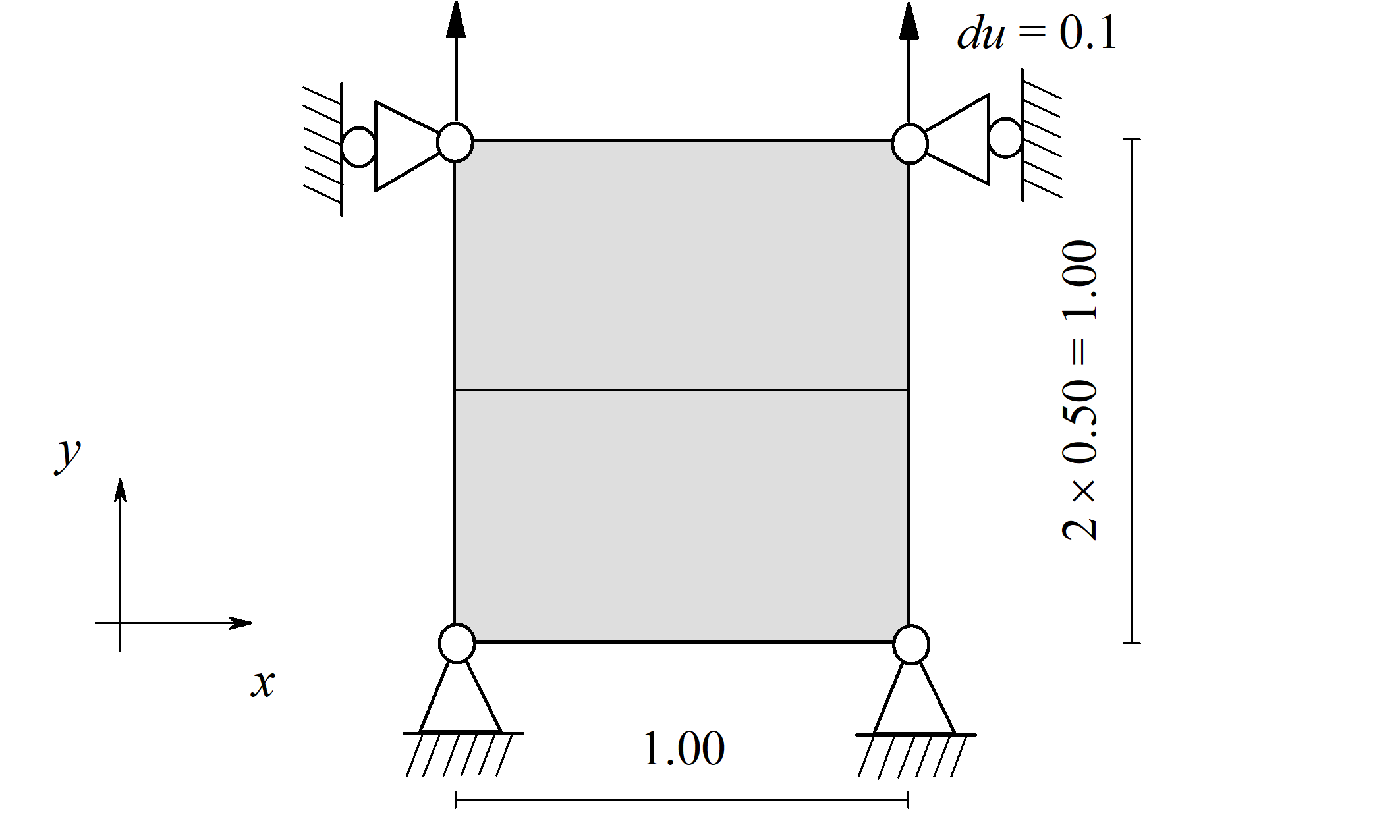## Fracture modeling with phase field method

1. Simple tension with 2 elements

The tutorial presents a simple conversion between the input file generated by ABAQUS and the use of the new UEL.

The instructions can be downloaded from here. While the files used and created through the tutorial are accessible from this link.

.2. Single edge notched sample & MATLAB converter

The tutorial presents a single edge notched sample and how to develop simple models using ABAQUS/CAE and convert them with a MATLAB script (still in Beta testing stage).

It contains a FORTRAN script with both triangular and square elements. The instructions are shown here. While the files used and created through the tutorial are accessible from this link.

.
3. 3D cracked cylinder & MATLAB converter in 3D

The tutorial presents a simple cylinder with a sharp crack with the 3D MATLAB converter script (still in Beta testing stage).

It contains a FORTRAN script with both tetrahedral and hexahedral elements. The instructions are shown here. While the files used and created through the tutorial are accessible from this link.

.
4. Anisotropic/asymmetric energy degradation & Multiple material definition

The tutorial demonstrates the different stress response as a function of asymmetric energy release. Additionally it presents a example to define multiple materials in a single part.

The uploaded package contains the FORTRAN script with both triangular and square elements with the converting MATLAB script. The detailed video is posted here. While the files used and created through the tutorial are accessible from this link.

.

Implementation details are summarized here.

5. Contact simulation & MATLAB converter

The tutorial presents an idealized Brazilian test to demonstrate the way to define contacts and forces with the phase field UEL.

The instructions are shown here. While the files used and created through the tutorial are accessible from this link.

.
6. Built-in material models in ABAQUS with the phase field UEL

The tutorial demonstrates how to use built-ni material models in ABAQUS next to the UEL phase field elements.

The uploaded package contains the updated MATLAB converter script. The detailed video is posted here. While the files used and created through the tutorial are accessible from this link.

.
7. Dynamic fracture & MATLAB converter update

The tutorial presents a dynamic fracture simulation using the phase field UEL.

The instructions are shown here. While the files used and created through the tutorial are accessible from this link.

.
8. Automatic time integration

The tutorial presents the automatic time integration developed for the phase-field fracture in ABAQUS.

The instructions are shown here. While the files used and created through the tutorial are accessible from this link.

.
9. Linear damage function (AT1) in ABAQUS

This tutorial presents a simulation in ABAQUS/CAE using a UEL/UMAT phase-field option with a linear gradient geometric function, with the so-called AT1 model.

The instructions are shown here. While the files used and created through the tutorial are accessible from this link.

.